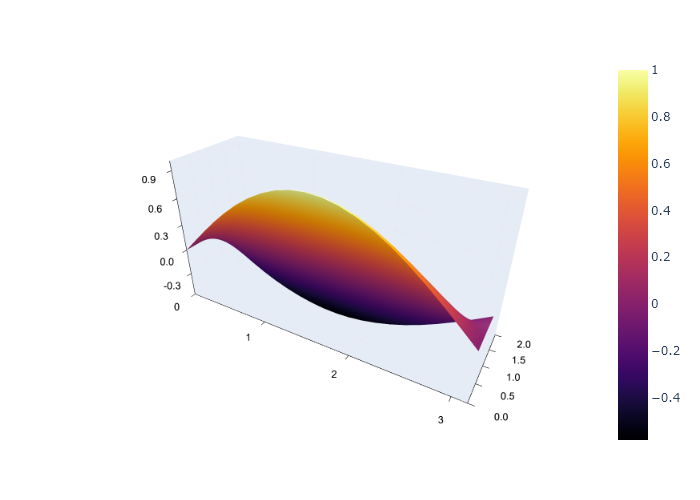# Fractional Order Partial Differential Equations

Warning

Supports for FPDE are still WIP......

Several models of physical and biological processes are better described using fractional PDEs than the corresponding integer-order PDEs. They serve as a generalization of the integer-order PDEs and give some degree of freedom in varying the rate of change of these physical and biological processes.

FractionalDiffEq.jl has support for solving fractional partial differential equations. Let's see an example here:

Time fractional differential equation:

$$$_{0}^{C}\!D_{t}^{\alpha}y - \frac{\partial^\beta y}{\partial |x|^\beta} = f(x,t)$$$

With initial and boundry conditions:

$$$y(0,t) = 0, \quad y(1,t) = 0 \qquad \quad y(x, 0) = 0$$$

We can use the FPDEMatrixDiscrete algorithm to solve this problem:

using FractionalDiffEq, Plots

α = 0.7
β = 1.8
κ = 1
T = 2
m = 21
n = 148

result = solve(α, β, κ, T, m, n, FPDEMatrixDiscrete())

XX, YY = meshgrid(0.05^2/6 .*(0:147), 0:0.05:1)

plotlyjs()

plot(XX, YY, result, st=:surface)## Time fractional diffusion equation

When we are modeling our problems, time fractional derivative maybe more suitable for our model, which changes the diffusion equation to Diffusion equation with time fractional derivative

$$$^C_0D^\alpha_tu=\kappa\frac{\partial^2u}{\partial x^2}$$$$$$y(0,t) = 0, \quad y(1,t) = 0 \qquad \quad y(x,0) = \sin(x)$$$

Here, $\kappa$ is the diffusion coefficient

The solution of this Diffusion equation is given as:

$$$u(x, t) = E_\alpha(-t^\alpha)\sin(x)$$$

Here $E_\alpha(-t^\alpha)$ is the Mittag Leffler function.

using FractionalDiffEq

K = 1
α = 1.9
dx = pi/20
dt = 0.01
n = 2
xStart = 0
xEnd = pi
u0t = 0
uendt = 0
u0(x) = sin(x)

using Plots
plotlyjs()
U=solve(α, dx, dt, xStart, xEnd, n, K, FiniteDiffEx())
plot(x, t, U, st=:surface)## Fractional partial differential equations with time delay.

As the generalization of DPDE, FDPDE is also an interesting topic in fractional differential equations.

To solve FDPDE problem with FractionalDiffEq.jl, we need to define our FDPDEProblem.